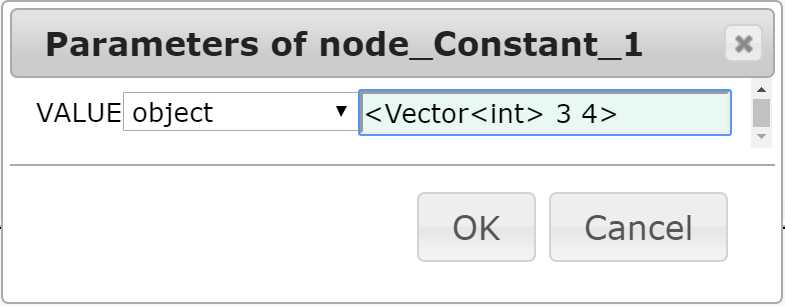## 4.2.1 Vector

Vector is a data type used to store an array of data. No matter what data is stored in Vector , it generally uses delegates such as Vector< Obj > with ObjectRef  as an element, and Vector< int > , Vector< float > that has an element with values (int , float ). Since the values are always paired before being used, assigning angles in ConstantLocalization , or expressing the output of localization results in LocalizeMUSIC can be executed. Below is the definition of a Vector data type. Base Vector is a data type that implements the method for HARK  . As shown below, the Vector inherited vector  type of STL.

template<class T> class Vector :
public BaseVector, public std::
vector<T>


The ToVect  node in the Conversion  category accepts input in int , float , etc., and then converts and output the value into Vector . In addition, to use Vector as a parameter of a node, the data type of the parameter must be set to Object , and then the values must be set to text in the same pattern below. For example, to use two int elements 3 and 4 as a parameter, the text should be entered as shown in Figure 4.1. However, one should be careful not to put space after the first <  before writing Vector .Figure 4.1: Input example of Vector